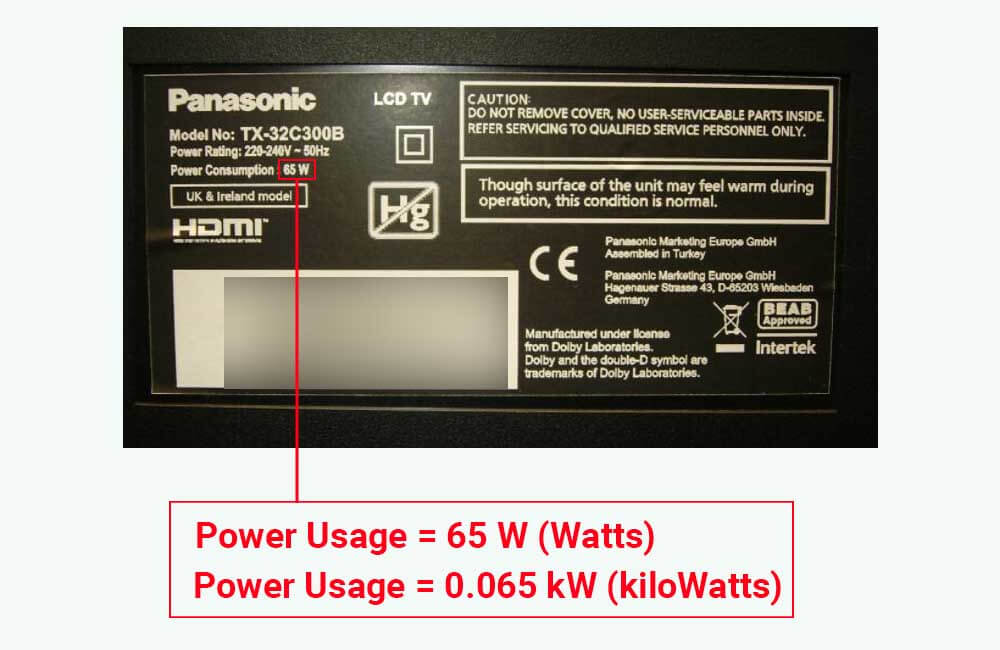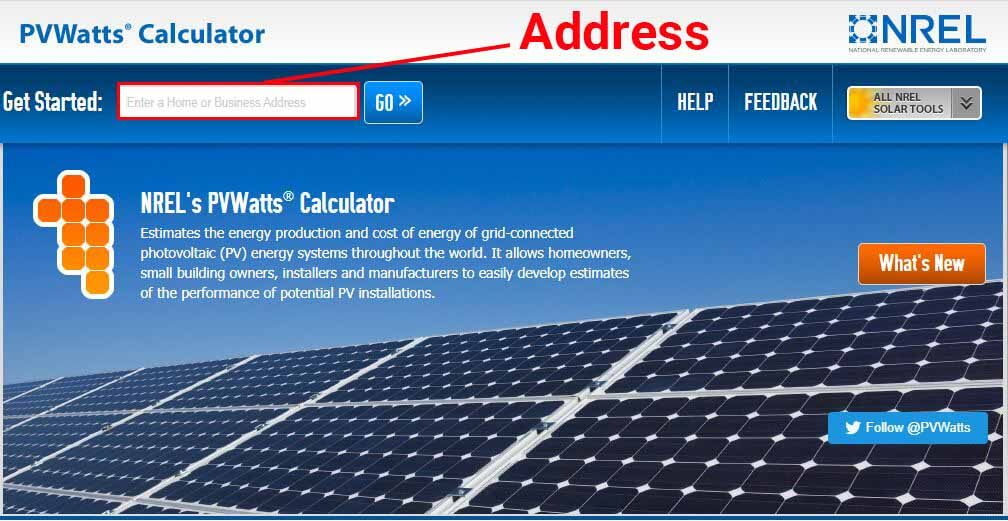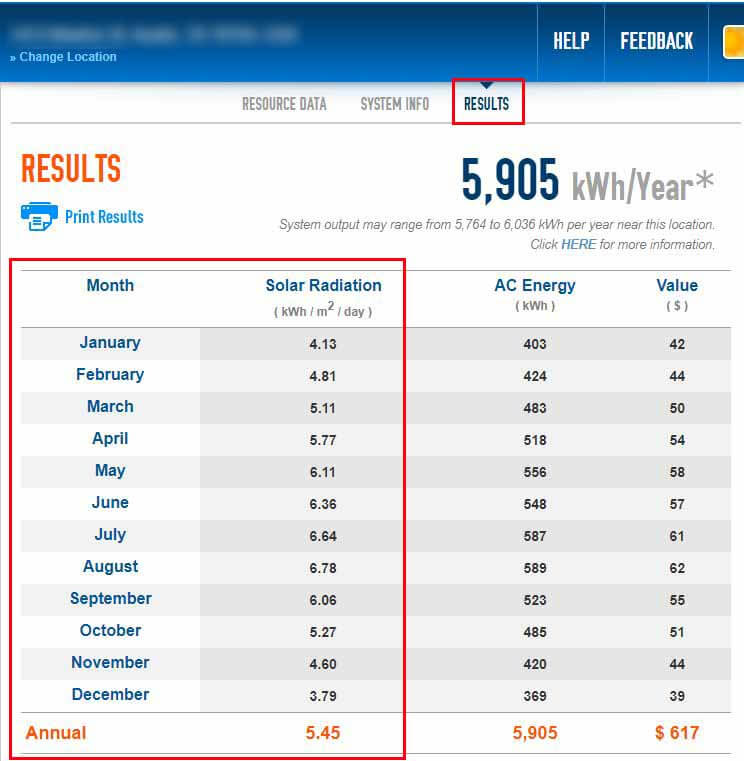# kW vs kWh: What is the difference between Power and Energy?

Electricity measurement units can be very confusing and are often misunderstood, this is mainly because electricity itself is a complicated subject.

In my article about Electricity and its measurement units, I discuss Amps, Volts, Watts, and Watt-hours.

In this article, I’ll explain what kW and kWh are, what they represent and measure, and what the difference between them is.

## kW vs kWh: What is the difference?

The terms “kW” and “kWh” are often used interchangeably, but they refer to two different aspects of electricity.

“kW” stands for “kiloWatt”, which is equal to 1000 Watts, and “Watts” is the conventional unit for measuring “Electrical Power”. On the other hand, “kWh” stands for “kiloWatt-hour”, which is equal to 1000 Watt-hours, and “Watt-hours” is the conventional unit for measuring “Electrical Energy”.

1 kiloWatt (kW) = 1000 Watts (W)

1 kiloWatt-hours (kWh) = 1000 Watt-hours (Wh)

But this still doesn’t explain the difference, does it?

Let me explain.

### What is the difference between Electrical Energy (kWh) and Electrical Power (kW)?

Well, the difference is this:

While Energy, measured in Wh or kWh, represents the “quantity” of electricity that has been consumed or produced over a certain period of time, Power, measured in W or kW, represents the “rate” at which Energy is consumed or produced at a given moment.

In other words, Power is instantaneous, Energy is accumulative, and Power is the rate at which Energy is being accumulated.

Your utility bill shows your electricity usage in kWh (kiloWatt-hours) or Wh (Watt-hours) and not in kW (kiloWatt) or W (Watts) because you pay for the quantity of electricity you’ve consumed during your billing period and not the rate at which you’ve been consuming electricity.

Unless it’s about battery storage capacity, whenever Energy (kWh) is spoken of, time should always be mentioned.

For instance, if someone says that an air conditioner consumes 10 kWh (10,000 Wh) of Energy, the next question that should come to mind is: “over how much time?”.

The correct way to talk about the Energy consumption of the air conditioner would be to say “the air conditioner consumes 10 kWh per day”, or “the air conditioner consumes 300 kWh per month”.

On the other hand, you could say that – at a given moment – an air conditioner uses 1.5 kW (1500 W) of Power, but you can’t say that an air conditioner uses 1.5 kW per day.

To understand this even better, let’s look at a common analogy used to describe the difference between Power (kW) and Energy (kWh).

### kW vs kWh: The Water Analogy

To understand the difference between “kW” and “kWh”, think of a bucket being filled with water.

In this analogy, the rate at which the water is flowing represents Electrical Power (kW), and the quantity of water in the bucket represents Electrical Energy (kWh).The higher the flowing rate of the water, the quicker the bucket fills up, and likewise, the higher the Power that is being used or produced, the faster Energy is consumed or produced.

### What is the relationship between Energy (kWh) and Power (kW)?

Since Power is the rate at which Energy is consumed or produced, the relationship between Power and Energy is expressed as such:

Energy (kWh) = Power (kW) x Time (hours)

or

Energy (Wh) = Power (W) x Time (hours)

This means that if you know the power usage of an appliance in (Watts or kiloWatts), and the amount of time for which the appliance has been used (in hours), you could calculate the amount of energy that the appliance has consumed over that amount of time (in Watt-hours or kiloWatt-hours).

Let’s look at an example.

## Your appliances’ Power usage (kW) vs their Energy consumption (kWh)

Appliances usually come with a nameplate, a specification label, or some sort of documentation that specifies their Power usage or “Wattage”. For example, the following image is of a specification label on the back of a 32″ TV:The manufacturer specifies that the TV uses 65 Watts of Power when it’s ON, which is equivalent to 0.065 kW of power. Using this piece of information, we could calculate the Energy consumption of the TV over a certain period of time.

Related: How many Watts does a TV use?

For example, let’s say that we want to calculate the monthly energy consumption of the TV, and let’s assume that – on average – the TV is on for 4 hours a day.

We could first calculate the daily energy consumption of the TV as such:

Daily Energy Consumption (in Watt-hours) = Power Usage (in Watts) x Daily Usage Duration (in hours)

Daily Energy Consumption (in Watt-hours) = 65 Watts x 4 hours

Daily Energy Consumption (in Watt-hours) = 260 Watt-hours

To determine the monthly energy consumption of the TV, we simply multiply its average daily energy consumption by 30 (the number of days in a month):

Monthly Energy Consumption (in Watt-hours) = Daily Energy Consumption (in Watt-hours) x 30

Monthly Energy Consumption (in Watt-hours) = 260 Watt-hours x 30

Monthly Energy Consumption (in Watt-hours) = 7800 Watt-hours

This is the energy consumption in Watt-hours (Wh), to determine the energy consumption in kiloWatt-hours (kWh), we simply divide by 1000:

Monthly Energy Consumption (in kiloWatt-hours) = Monthly Energy Consumption (in Watt-hours) ÷ 1000

Monthly Energy Consumption (in kiloWatt-hours) = 7800 Wh ÷ 1000

Monthly Energy Consumption (in kiloWatt-hours) = 7.8 kWh

If you’re trying to estimate the energy consumption of your appliances, I recommend using this Energy Consumption Calculator that I put together. The calculator will especially be helpful if you don’t know the power usage of your appliances.

Now, let’s discuss the difference between power and energy in solar panels.

## kW vs kWh in solar

Solar panels are rated in units of Electrical Power (Watts and kiloWatts), for instance, a single solar panel could be rated at 300 Watts (0.3 kW) of power, and a whole solar installation could be rated at 6000 Watts (6 kW) of power.

As explained above, Power is the rate at which Energy is consumed or produced. In the case of solar panels, the power rating (W or kW) of a solar panel or system indicates the rate at which the solar panel or system is capable of producing Energy (Wh or kWh).

For example, if a solar panel is rated at 300 Watts (0.3 kW), it means that the solar panel is capable of producing 300 Watts or 0.3 kW of power in the “right conditions”.

If the “right conditions” are provided, and the 300W solar panel produces 300 Watts or 0.3 kW of Power continuously for 1 hour, it will have produced 300 Watt-hours (Wh) or 0.3 kiloWatt-hours (kWh) of Energy by the end of that hour.

If the 300W solar panel produces 300 Watts (0.3 kW) of Power continuously for 3 hours, it will have produced 900 Watt-hours (Wh) or 0.9 kiloWatt-hours (kWh) of Energy by the end of those 3 hours.

But, what are the “right conditions”?

The answer is: Mainly, the right amount of sunlight.

However, the right amount of sunlight is not always available.

### Power Production of a solar panel (kW): Standard Test Conditions vs Actual Operating Conditions

Standard Test Conditions, or “STC” for short, refer to the industry standard under which solar panels are tested and given a Power Output rating. These standardized test conditions ensure that solar panels from different manufacturers can be compared accurately.

STCs include a few conditions, but for the sake of simplicity, we’ll only focus on the one that matters the most: Solar Irradiance

Solar Irradiance can be defined as the amount of sunlight hitting a certain surface area and is typically measured in Watts per square meter (Watts/m²).

When testing solar panels, along with other Standard Testing Conditions, a standard Solar Irradiance of 1000 watts/m² (1 kW/m²) is used to simulate the typical peak sun conditions found on a clear day around noon. The amount of Power that a solar panel produces under this exact amount of “artificial sunlight” becomes the Power Rating of the solar panel.

In other words, a 300W solar panel would only produce 300 Watts of power at its output, at a given moment, if these conditions or similar conditions are met.

But this doesn’t mean that the solar panel needs these exact conditions to produce power and generate energy, it just means that solar panels do not always produce their rated output.

For instance, this is what the amount of sunlight, or Solar Irradiance, that a solar panel receives during your typical sunny day looks like:In general, the amount of power that a solar panel produces is proportional to the amount of sunlight that it receives (Solar Irradiance).

For example, consider a solar panel rated at 300 Watts of power. Under the same Solar Irradiance conditions as the ones shown in the image above, the Power Output of a 300W solar panel would look like this:At 10 AM, you can see that our 300W solar panel only produces about 100 Watts (0.1 kW), which is only a third of its rated power (300 Watts). This is because at 10 AM, the amount of sunlight that the solar panel receives is still low, and the Solar Irradiance is only about 300 W/m².

The fact that the power output of solar panels is proportional to Solar Irradiance, is precisely why a solar panel produces the most amount of power at noon, when sunlight intensity is at its highest, and doesn’t produce any power at night, when there is no sunlight.

Now that we’ve discussed the power ratings of solar panels, and their power production, let’s talk about their energy production.

### Energy Production of a solar panel (kWh): Explaining Peak Sun Hours

As explained above, the amount of power that a solar panel produces at a particular moment depends on the amount of “sunlight power” that it receives at that particular moment. This “sunlight power” is measured in Watts per square meter (W/m²), and is referred to as “Solar Irradiance”.

Similarly, the amount of energy that a solar panel generates over an hour, a day, or a month, depends on the amount of “sunlight energy” that it received over that hour, day, or month.

This “sunlight energy” is measured in kiloWatt-hours per square meter (kWh/m²), and is referred to as “Peak Sun Hours”.

#### So, what is a Peak Sun Hour?

Peak Sun hours can be defined as the amount of “sunlight energy” per unit area, that a certain area receives over a certain period of time.

For example, let’s say that an area receives 1000 Watts/m² (or 1 kW/m²) of sunlight continuously for 5 hours, the same area would have received 5000 Watt-hours/m² (or 5 kWh/m²) of “sunlight energy” by the end of those 5 hours, and it could be said that the area received 5 Peak Sun Hour in those 5 hours.

However, this is just a simplified example to explain the significance of Peak Sun Hours. As explained above, Solar Irradiance does not remain constant, especially not for 5 hours.

So, in reality, Peak Sun Hours are measured by tracking Solar Irradiance, typically, over the course of a day.

For instance, in the summer when the sun rises earlier in the morning and sets later in the evening, and direct sunlight is generally abundant, a certain area might receive up to 8000 Wh/m² (8 kWh/m²) of “sunlight energy” over the course of a day, which is equivalent to 8 Peak Sun Hours.

In the winter, when the sun rises later in the morning and sets earlier in the evening, and direct sunlight is generally scarce, the same area might only receive 3000 Wh/m² (3 kWh/m²) over the course of a day, which equates to 3 Peak Sun Hours.

#### Now, what do Peak Sun Hours have to do with the energy production of solar panels?

Peak Sun Hours are a measure of the amount of solar energy that is available for solar panels to convert into energy. The relationship between Peak Sun Hours, and the energy production of solar panels, can be expressed as follows:

Energy Production of the solar panel (kWh) = Power Rating of the solar panel (kW) x Peak Sun Hours

For example, consider a 300W (0.3kW) solar panel that, on average, receives 5 Peak Sun Hours per day. The average daily energy production of the solar panel can be calculated as such:

Daily Energy Production (kWh) = Power Rating of the solar panel (kW) x Daily Peak Sun Hours

Daily Energy Production (kWh) = 0.3 kW x 5 Peak Sun Hours

Daily Energy Production (kWh) = 1.5 kWh

Now, let’s say that we’re trying to determine the Power rating (kW) of a solar panel that could offset a certain amount of Energy consumption (kWh). In this case, the following formula is used:

Power Rating of the solar panel (kW) = Energy Consumption (kWh) ÷ Peak Sun Hours

For instance, let’s say I’m trying to determine the amount of solar power needed to run a refrigerator, and let’s also assume that I know the following:

• My refrigerator uses 2 kWh of energy on a daily basis
• In my area, the solar panel(s) would – on average – receive 5 Peak Sun Hours per day.

The size of the solar panel that I need to run my refrigerator can be calculated as such:

Power Rating of the solar panel (kW) = Daily Energy Consumption of the Refrigerator (kWh) ÷ Daily Peak Sun Hours

Power Rating of the solar panel (kW) = 2 kWh ÷ 5 Peak Sun Hours

Power Rating of the solar panel (kW) = 0.4 kW

So, based on the daily energy consumption of my refrigerator, and the daily Peak Sun Hours that I get, I would need 0.4 kW or 400 Watts of solar panels.

Now, this leads to an important question: How do you determine the Peak Sun Hours that you get?

#### How to determine the Peak Sun Hours in your location?

There are a few resources online where you can get some estimates of the Peak Sun Hours in your location. The best one, in my opinion, is the PVWatts Calculator by NREL (National Renewable Energy Laboratory).

Using historical weather data, the PVWatts calculator is able to estimate the daily Peak Sun Hours in your location.

To use the tool, follow these 2 steps:Once you’ve submitted your location to the calculator, go to the “Results” section of the tool to access the data:As explained above, “Peak Sun Hours” is just another way of saying kiloWatt-hours per square meter (kWh/m²). So, “Daily Peak Sun Hours” would be another way of saying “kWh/m²/day”.

In the “Results” section, and in the column that says “Solar Radiation in kWh/m²/day”, the calculator provides monthly and annual averages of the daily Peak Sun Hours in this location.

For example, in the image above, you can see that in the month of May, this location gets 6.11 Peak Sun Hours per day on average. But, in the month of December, this location only gets 3.79 Peak Sun Hours per day.

At the bottom, the calculator estimates that the annual average Peak Sun Hours in this location is 5.45 Peak Sun Hours per day.